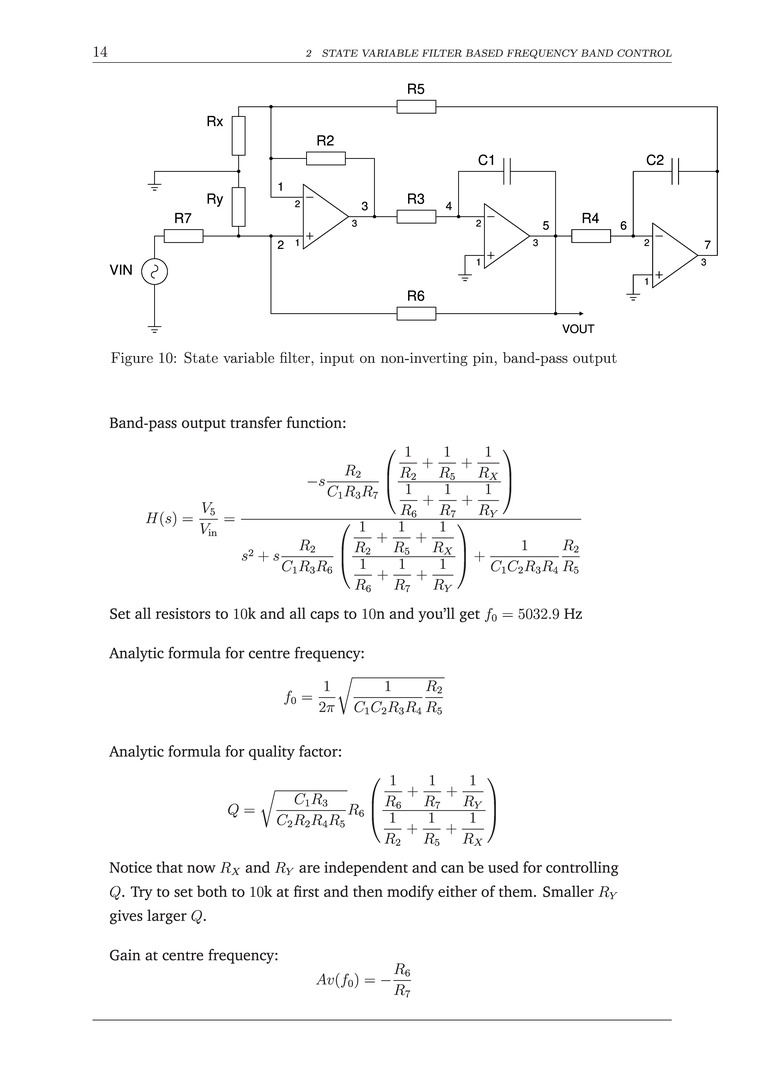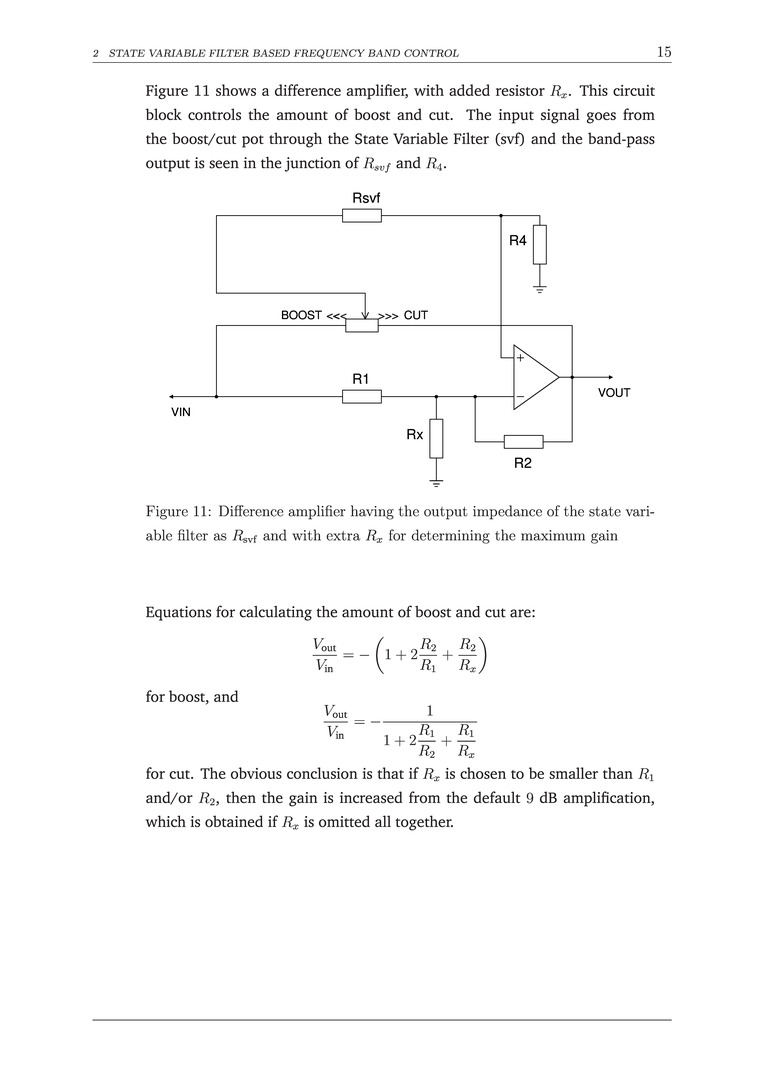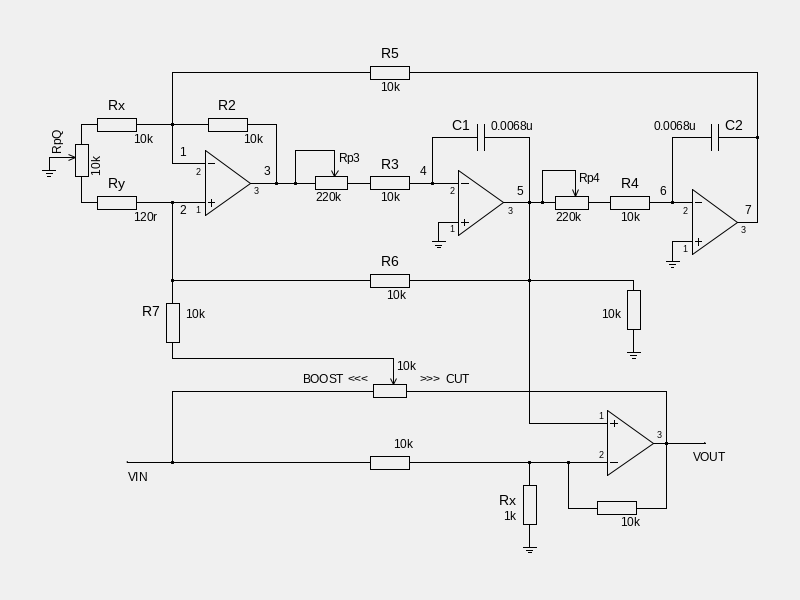Author Topic: Two band Parametric EQ  (Read 1940 times)

Guitar_Duderino

• member2
•• Posts: 26
• Total likes: 0Two band Parametric EQ
« on: April 17, 2019, 01:16:17 PM »
Salutations, all.

My head is spinning these days trying to understand these many options for parametric EQ circuits. These circuits can be various, as I understand from different webpages. Problem is that some give its equations, some do not, and for these I cannot figure its equations, with which I would adapt its components to my EQ's needs.

Before showing these different circuits and their pages I want your advice.

I want to build my two band parametric EQ (band: 50Hz - 2kHz, band: 500Hz - 20kHz, Q: 0.5 - 16, gain: ±20dB) with potentiometers for frequency/Q/gain for each band.

How should I do this? Do you already know some solution for this?

Many thanks for your help and time.

MrStabRe: Two band Parametric EQ
« Reply #1 on: April 17, 2019, 01:29:04 PM »
hi Duderino,

IMO your best choice is a state-variable filter circuit. i make and sell these all the time, thanks to the assistance of people on this forum a few years back.

basic overview here:
http://sound.whsites.net/articles/state-variable.htm

I don't have all the equations with me atm, but if you like i could figure out the component values you need for each parameter you specified, and send them over in a day or 2. if someone wants to jump in sooner, though, go for it!

-g

slacker

• Poster2
•• Posts: 6331
• Total likes: 211
• Ian M. - EnglandRe: Two band Parametric EQ
« Reply #2 on: April 17, 2019, 02:02:10 PM »
There's a relatively simple parametric EQ design here, including formulas for calculating the frequencies and things  http://www.geofex.com/Article_Folders/EQs/paramet.htm

Guitar_Duderino

• member2
•• Posts: 26
• Total likes: 0Re: Two band Parametric EQ
« Reply #3 on: April 17, 2019, 03:00:16 PM »
There's a relatively simple parametric EQ design here, including formulas for calculating the frequencies and things  http://www.geofex.com/Article_Folders/EQs/paramet.htm

Thank you, Slacker. I already went through that page and did not fully understand it... That parametric EQ circuit is for two bands, right? And on that paragraph

Quote
Some good places to set the frequency bands for these controls are from about 100 Hz at a minimum up to several kHz. We only get about a 4.5:1 range, so we can pick some values. If we use C1= 0.15uF and C2 = 0.02uF, we get a range of about 100Hz to 450Hz

how did he get those 100Hz? He gave equations with C2 and here where does C1 enter?

MrStabRe: Two band Parametric EQ
« Reply #4 on: April 17, 2019, 03:50:33 PM »
the gyrator approach, as shown in the Geofex link, is definitely more straightforward to build, but the gain and Q change as the Frequency pot is turned. State-variable uses more parts, but is constant Q and gain.

Guitar_Duderino

• member2
•• Posts: 26
• Total likes: 0Re: Two band Parametric EQ
« Reply #5 on: April 17, 2019, 05:08:16 PM »
And I want that...all independent.

basic overview here:
http://sound.whsites.net/articles/state-variable.htm

which I had seen, below Figure 1 he says f0 = 1/(2*π*R*C), which with those components would be 1/(2*π*10000*0.00000001)? This gives 1592.35Hz and he says 159Hz. How is this?

MrStabRe: Two band Parametric EQ
« Reply #6 on: April 17, 2019, 05:31:58 PM »
i think you have one zero too many in your equation, it should be 0.0000001 farads

Guitar_Duderino

• member2
•• Posts: 26
• Total likes: 0Re: Two band Parametric EQ
« Reply #7 on: April 17, 2019, 05:39:21 PM »
Certainly that would solve it, but is that not 100nF (in Figure 1 C=10nF)?

MrStabRe: Two band Parametric EQ
« Reply #8 on: April 17, 2019, 08:04:16 PM »
ah, i thought you meant Fig 5. yes, i think that is an error.

antonisRe: Two band Parametric EQ
« Reply #9 on: April 18, 2019, 05:34:20 AM »
When dealing with too many zeros after decimal point it's more convenient to use micro(μ) & mega(Μ) values in case they're involved in a product..
(e.g. for 16Hz with 100k & 100nF, use 0.1 for resistor & 0.1 for capacitor..)
but I like what follows:
"put the coffee down, Antonis, and nobody will get hurt."
"Arggg, Jim ...."

ElectricDruidRe: Two band Parametric EQ
« Reply #10 on: April 18, 2019, 03:57:22 PM »
the gyrator approach, as shown in the Geofex link, is definitely more straightforward to build, but the gain and Q change as the Frequency pot is turned. State-variable uses more parts, but is constant Q and gain.

Not only that, but the gyrator approach really does only give a fairly limited frequency range, and the OP wanted a 1:40 range. That means a state variable or something else with similar properties is the only option.

antonisRe: Two band Parametric EQ
« Reply #11 on: April 19, 2019, 05:48:06 AM »
IMHO, for such a wide frequency spectrum, a 3 band parametric EQ should be more appropriate choice..
(by narrowing range you can be more flexible in Q setting and cap/res values..)
but I like what follows:
"put the coffee down, Antonis, and nobody will get hurt."
"Arggg, Jim ...."

ElectricDruidRe: Two band Parametric EQ
« Reply #12 on: April 19, 2019, 07:12:33 AM »
IMHO, for such a wide frequency spectrum, a 3 band parametric EQ should be more appropriate choice..
(by narrowing range you can be more flexible in Q setting and cap/res values..)

Yes, perhaps you're right. It's certainly true that the original specification of 1:40 range on the frequency control and 1:32 range on the resonance control is "demanding"Reducing those requirements to something less extreme would make the thing easier to design, and also have the benefit of making the controls much less twitchy. Imagine trying to tune the frequency to notch out feedback or something when a fraction of a degree might make a 1000Hz difference in frequency...not going to work.

Guitar_Duderino

• member2
•• Posts: 26
• Total likes: 0Re: Two band Parametric EQ
« Reply #13 on: April 22, 2019, 04:52:32 PM »
Please bear with me while I try to understand and solve this.

I think this circuit could solve my EQ needs.(Full circuit: http://byocelectronics.com/parametricEQschem.pdf)

This circuit was made with three bands and here is one band shown.

Are

fmax = 1/(2*π*R15*C5)
fmin = R10/(2*π*VR3b*R15*C5)

?

(This is by comparison with this circuit (https://damien.douxchamps.net/elec/param_eq/), in which I see some similarity for frequency, but not for Q and boost-cut.)

What equations here for frequency/Q/boost-cut?
« Last Edit: April 23, 2019, 08:49:35 AM by Guitar_Duderino »

ElectricDruidRe: Two band Parametric EQ
« Reply #14 on: April 22, 2019, 06:11:46 PM »
Are

fmax = 1/(2*π*R15*C5)
fmin = R10/(2*π*VR3b*R15*C5)

?

Yes, it certainly looks like it. Note that the difference between those two equations is the ratio R10/VR3b, which in the BYOC circuit is 1.6/10=0.16, give roughly 1:6 range. In the other circuit it's 39R/10K which gives a range of over 250:1! So it really should cover the 40Hz to 10KHz they claim.

Quote
What equations here for frequency/Q/boost-cut?

Sorry, I don't know. There are some for standard state-variable filters, but that one alters the way the frequency is varied (but we've got the equations for that bit, it seems), and I've not seen the Q handled like that before either.
I notice the damien.douxchamps.net provides a SPICE model - that's another approach. Experiment in SPICE until you get it how you want it?

Guitar_Duderino

• member2
•• Posts: 26
• Total likes: 0Re: Two band Parametric EQ
« Reply #15 on: April 23, 2019, 08:11:16 AM »
Thank you, ElectricDruid. Still it would be great, if someone fresh from Circuit Analysis could help on these equations.

In this circuit there are many resistors with same value (5.1kΩ and 1.6kΩ). If one is changed, should all others be changed? (Damien Douxchamps thus seems to indicate.)

And BYOC says that this circuit gives
33Hz - 330Hz,
250Hz - 2.5kHz,
1.1kHz - 11kHz,
(1:10 ratio).
But with these equations we get
50Hz - 312Hz,
416Hz - 2.6kHz,
1.8kHz - 11.5kHz.
Should these be so?

To attain that 1:10 ratio either R10 needed to be 1kΩ or these equations lack something.
« Last Edit: April 23, 2019, 08:50:41 AM by Guitar_Duderino »

jatalahd

• member2
•• Posts: 80
• Total likes: 21
• Jarmo L. - FinlandRe: Two band Parametric EQ
« Reply #16 on: April 23, 2019, 10:04:29 AM »
Hi Duderino,

I did some math work for you. It is not directly for the circuit you posted a schematic, but it comes close. You might get something out of it. I analysed the system in two parts, firstly the equations for the state variable filter with Q-control resistors, Rx and Ry:It produces the band-pass filtering with variable frequency. Unfortunately it would take too much effort to evaluate the frequency control as it is done in your referenced schematic. Here you can just vary R3 and R4 simultaneously, just calculate the centre frequency from the provided formulas.

Then the difference amplifier produces the boost/cut amplification according to the given equations:Again this is not 1 to 1 match with your schematic, but it does what you want it to do when combined with the state variable filter.

Hopefully this gets you forward. You need to improvise a little and do some own homework, but that is often required anyway.

jatalahd

• member2
•• Posts: 80
• Total likes: 21
• Jarmo L. - FinlandRe: Two band Parametric EQ
« Reply #17 on: April 23, 2019, 02:15:44 PM »
Hi again,

I got carried away  and made a SPICE simulation. This is the complete circuit that will meet your requirement of the lower band (from about 100 Hz to 2kHz, Q from 0.5 to 16 and gain +-20dB):Note that this is only a simulated model, the real circuit might need some additional resistors here and there to keep the circuit stable.

jatalahd

• member2
•• Posts: 80
• Total likes: 21
• Jarmo L. - FinlandRe: Two band Parametric EQ
« Reply #18 on: April 25, 2019, 05:04:23 AM »
Are

fmax = 1/(2*π*R15*C5)
fmin = R10/(2*π*VR3b*R15*C5)

?

I did some calculations for these also. The equation for fmax is correct and can be used as is for BYOC circuit and similar circuits for example in Rod Elliots pages. The correct equation for fmin is

fmin = (R15||R10||VR3b)/(2*π*VR3b*R15*C5), and it gives 33 Hz for the low band minimum frequency in BYOC circuit

The R15||R10||VR3b is the parallel resistance of R15, R10 and VR3b. This can be calculated as R15||R10||VR3b = 1/(1/R15 + 1/R10 + 1/VR3b). In Damien Douxchamps the 39 ohm resistor is so small, that the parallel resistance equals the value of that resistor.

ElectricDruidRe: Two band Parametric EQ
« Reply #19 on: April 25, 2019, 05:31:25 PM »
Nice work, Jarno!